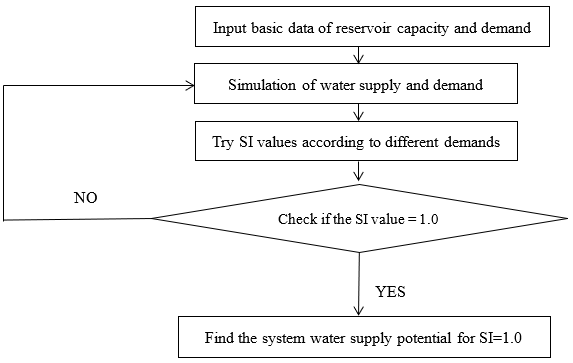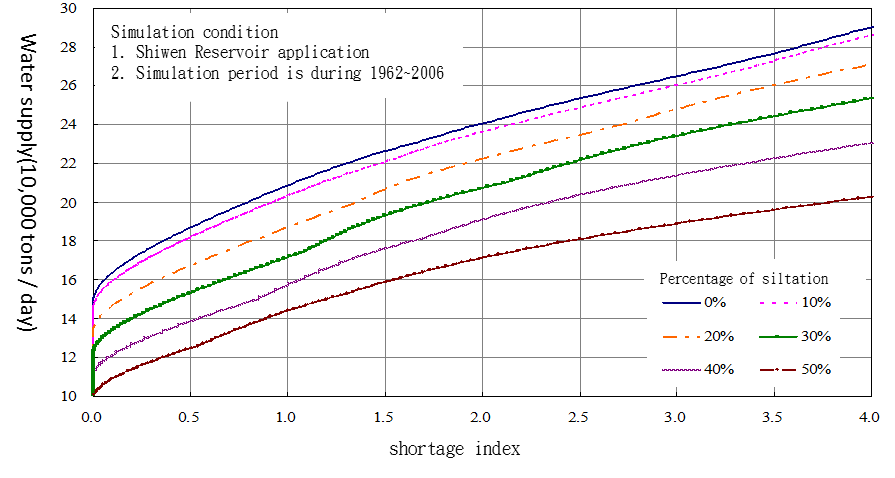﻿ Water Resources Planning Institute, WRA, MOEA-Reservoir Supply Potential Estimation
1. ## Reservoir water supply potential assessment and simulation principle

To assess the water supply capacity of a water resource system, we generally use shortage index (SI) to evaluate the water supply potential. In Taiwan, the general standard of water shortage index value is 1.0. The calculation process is shown in Figure 1.Figure.1 Process chart of reservoir water supply potential calculation

2. ## Shortage Index

The Shortage Index (SI) was created by the US Army Corps of Engineers in 1975 to analyze the assessment of water shortage. Taiwan generally also uses SI to assess the risk of water shortage in tap water. The water shortage index is calculated as equation (1)：

`SI=100/N ∑_(i=1)^N ((DF_i)/D_i )^2 `(1)

In equation (1), SI = Shortage Index，N = Analysis years ，DFi = Water shortage in the i-th year of the simulation period，Di = Demand water in the i-th year of the simulation period.

3. ## The relationship between S.I. and water supply potential

According to the process chart, the water demands and corresponding water shortage indexes given by the trial and error method are plotted on the diagram. It can get the relationship between the water shortage index and the water supply potential, as shown in figure 2. In this example, the water supply potential of the reservoir is about 210,000 CMD when the water shortage index value is 1.0.Figure.2 the relationship between the water shortage index and the water supply potential